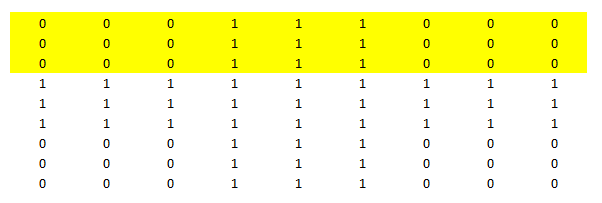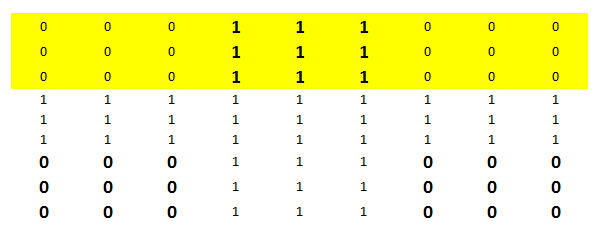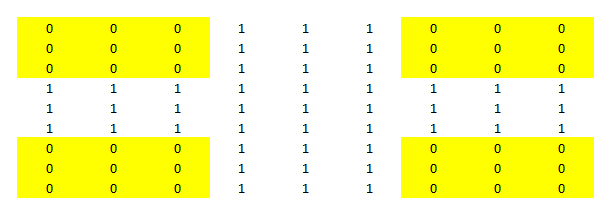Home » Getting smart with Machine Learning – AdaBoost and Gradient Boost

### Introduction

Machine Learning algorithms are like solving a Rubik Cube. You grapple at the beginning to figure out the hidden algorithm, but once learnt, some can even solve it in less than 7 seconds.

Suppose, you are stuck in following situation:

You are served with legion of data to generate useful insights. To nail this challenge, you got your best team members lined up or you come forward to lead from the front. You begin with building a predictive model. Then, you check the output statistics and gets disheartened. Reason being, the predictive power of the model is very low. Now, you desperately want to figure a way to increase the predictive power. What will you do?

There are some machine learning engines. These engines make use of certain algorithms and help user reach to the output stage. Some of the most popular engines are Decision Tree and Regression.

In this article, we’ll introduce you to some of the best practices used to enhance power of these engines to achieve a higher predictability using an additional booster.

These boosters are a type of ensemble technique. To learn more about them and other ensemble learning techniques in a comprehensive manner, you can enrol in this free course:  Ensemble Learning and Ensemble Learning Techniques

### Where are Boosted algorithms required?

Boosted algorithms are used where we have plenty of data to make a prediction. And we seek exceptionally high predictive power. It is used to for reducing bias and variance in supervised learning. It combines multiple weak predictors to a build strong predictor.

If you ever want to participate in Kaggle competitions, I would suggest that you bookmark this article. Participants in Kaggle completitions use these boosting algorithms extensively.

The underlying engine used for boosting algorithms can be anything. For instance, AdaBoost is a boosting done on Decision stump. There are many other boosting algorithms which use other types of engine such as:

1. GentleBoost

2. Gradient Boosting (Always my first choice for any Kaggle problem)

3.  LPBoost

4. BrownBoost

Perhaps, I can go on adding more engines to this list. But, I would like to focus on these five boosting techniques which are the most commonly used. Let’s first learn about – AdaBoost.

### What are Classifier Boosting Algorithms ?

Classification problem is the one where we need to assign every observation to a given set of class. The easiest classification problem is the one with binary class. This problem can be solved using AdaBoost. Let’s take a very simple example to understand the underlying concept of AdaBoost. You have two classes : 0’s and 1’s. Each number is an observation. The only two features available is x-axis and y-axis. For instance (1,1)  is a 0 while (4,4) is a 1. Now using these two features you need to classify each observation. Our ultimate objective remains the same as any classifier problem : find the classification boundary. Following are the step we follow to apply an AdaBoost.

Step 1 : Visualize the data : Let’s first understand the data and find insights on whether we have a linear classifier boundary. As shown below, no such boundary exist which can separate 0’s from 1’s.Step 2 : Make the first Decision stump : You have already read about decision trees in many of our previous articles. Decision stump is a unit depth tree which decides just 1 most significant cut on features. Here it chooses draw the boundary starting from the third row from top. Now the yellow portion is expected to be all 0’s and unshaded portion to be all 1’s. However, we see high number of false positive post we build this decision stump. We have nine 1’s being wrongly qualified as 0’s. And similarly eighteen 0’s qualified as 1’s.Step 3 : Give additional weight to mis-classified observations: Once we know the misclassified observations, we give additional weight to these observations. Hence, you see 0’s and 1’s in bold which were misclassified before. In the next level, we will make sure that these highly weighted observation are classified correctStep 4 : Repeat the process and combine all stumps to get final classifier : We repeat the process multiple times and focus more on previously misclassified observations. Finally, we take a weighted mean of all the boudaries discovered which will look something as below.#### More real life examples!

A classic use case where AdaBoost algorithms is in the problem of Face Detection. You can think of this as a more complex boundary detection as we found in last example. Once we have that boundary, we can now create features and classify if the image has a face or not.However, face recognition is commonly done after a gray scale transformation is done on the RCB image and finally a threshold is assumed to create face boundaries. You can read here to make transformation to gray scale or find threshold to create a black and white image. Once we have the transformation, we now analyze each patch of the image.

### Brief introduction to Regression boosters

Similar to classifier boosters, we also have regression boosters. In these problems we have continuous variable to predict. This is commonly done using gradient boosting algorithm. Here is a non-mathematical description of how gradient boost works :

Type of Problem – You have a set of variables vectors x1 , x2 and x3. You need to predict y which is a continuous variable.

Step 1 : Assume mean is the prediction of all variables.

Step 2 : Calculate errors of each observation from the mean (latest prediction).

Step 3 : Find the variable that can split the errors perfectly and find the value for the split. This is assumed to be the latest prediction.

Step 4 : Calculate errors of each observation from the mean of both the sides of split (latest prediction).

Step 5 : Repeat the step 3 and 4 till the objective function maximizes/minimizes.

Step 6 : Take a weighted mean of all the classifiers to come up with the final model.

We have excluded the mathematical formation of boosting algorithms from this article to keep the article simple.

### End Notes

Boosting is one of the most powerful tool used in machine learning. These models suffer the problem of over-fitting if the data sample is too small. Whenever the training sample is large enough, you should try boosting with many different engines as discussed in this article.

Were you haunted by any questions/doubts while learning this concept? Ask our analytics community and never let your learning process stop.

Have you used boosting before for any kind of analysis? Did you see any significant lift compared to the traditional models? Do let us know your thoughts about this guide in the comments section below.

### If you like what you just read & want to continue your analytics learning, subscribe to our emails, follow us on twitter or like our facebook page.

You can also read this article on our Mobile APP•Sam says:
•Gaurav says: